# Variance Ellipses

In this assignment, you'll continue working with current meter data. The main analysis tool we will learn are variance ellipses, a fundamental tool for analyzing velocity datasets. You'll see their relationship to the histogram on the (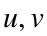) plane. Along the way you'll also learn a some more useful analysis and plotting functions in jLab.
Since now you know how Live Scripts work, feel free to just choose ‘Run All’ and then read through carefully. As before, you can add comments to yourself in your script about what you're learning or questions you have. You can also play around with modifying figures if you would like.

# Topics Covered

The topics covered in this assignment are
1. Rotating a complex-valued velocity
2. The direction of the mean flow is not the mean of the direction!
3. Importance of plotting velocities in a suitably rotated frame
4. More distrbutonal analysis using twodhist and twodstats
5. jLab plotting tools xtick and ytick, and hlines and vlines
6. Computing variance ellipses using jLab's specdiag
7. Plotting variance ellipses using jLab's ellipseplot
8. Plotting topography using jLab's topoplot
9. Setting a map's aspect ratio using jLab's latratio
10. Overlaying variance ellispes and bathymetry

# Data

For this assignment, we are going to use a mooring array from the Labrador Current on the west side of the Labrador Sea. The mooring is known as the ‘1244’ mooring. Please download my version of it here and put it on your Matlab search path. The data is publicly available here, though I am not aware of any paper analyzing it.
m1244
m1244 = struct with fields:
description: '1244 mooring 1994-1995'
creator: 'Created by the L_M1244 script by J.M. Lilly'
timestamp: '18-Oct-2018 05:40:00'
lat: 55.4786
lon: -53.6550
depths: [201 1001 1501 2751]
num: [7371×1 double]
p: [7371×4 double]
t: [7371×4 double]
cv: [7371×4 double]
You see that this is essentially the same structure as used in the ‘bravo94’ dataset, although here we do not have the rcm and cat substructures as there is only one type of instrument.
use m1244
provec(2,cv)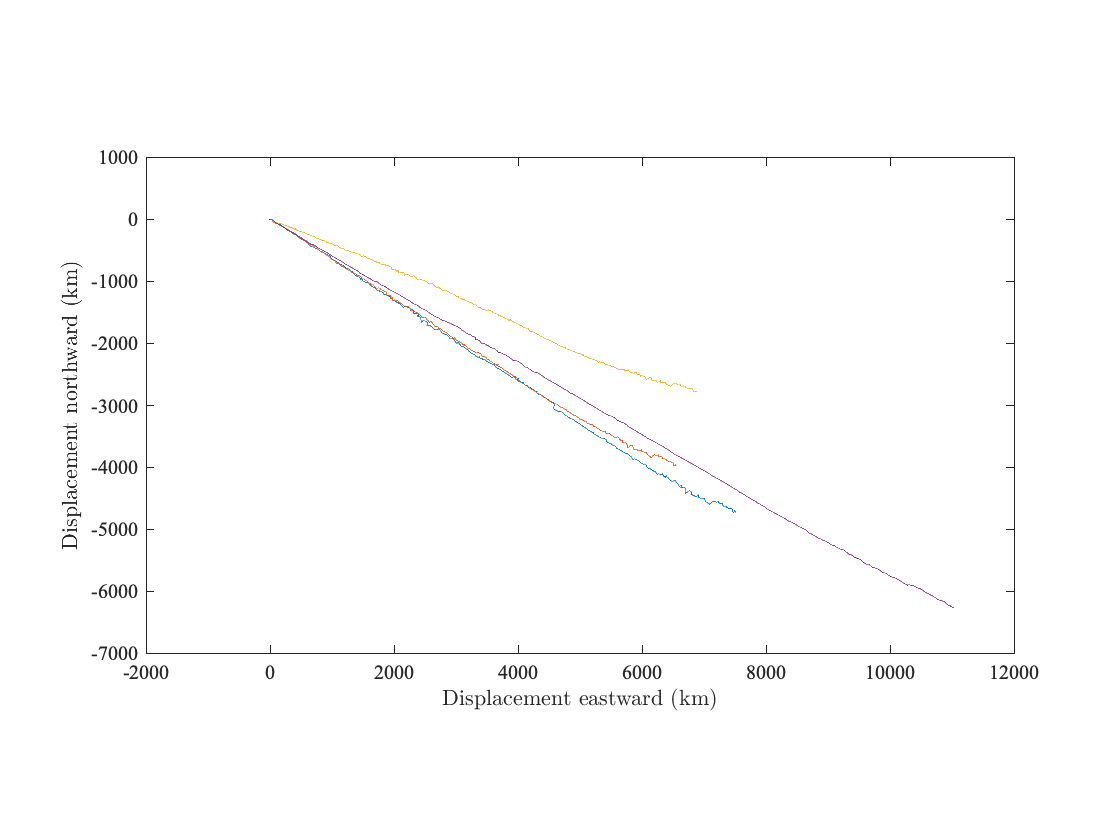Unlike the Bravo mooring, here there is a strong mean flow at all depths directed more or less to the southeast. Let's look at the deepest depth.
clf,
uvplot(yearfrac(num),cv(:,4)),axis tight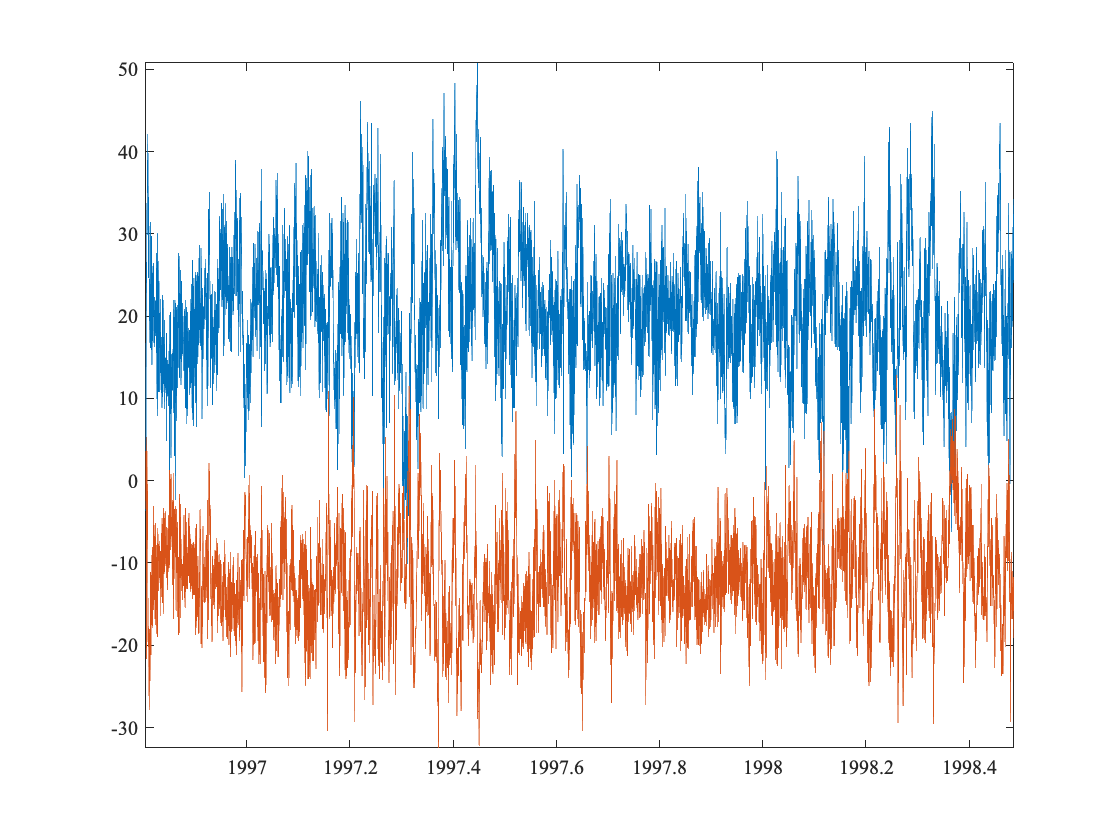The time series plot shows a great deal more small-scale variability than for the Bravo mooring. Note that the mean flow is apparent from this figure. The eastward (blue) component shows fluctuations about a positive mean, while the negative (orange) component shows fluctuations about a negative mean.
It is a little difficult to make sense of this plot, however, because it is not rotated into the most useful coordinate system.

# Rotating by the Mean Flow Direction

Next we will determine the direction of the mean flow, and rotate the currents so that u corresponds to the ‘downstream’ direction, while v corresponds to the ‘cross-stream’ direction. We will take a little time to do this so we understand how rotations work.
Firstly we find the direction of the mean flow at the deepest current meter, where the flow is strongest.
phi=angle(mean(cv(:,4)))
phi = -0.5167
phi*360/2/pi
ans = -29.6028
ans = -29.6028
Here rad2deg is a jLab function for converting radians to degrees, which essentially multiplies the angle by 360/(2π).
Rotation of complex-valued number z is straightforward. To rotate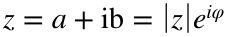through some angleϕ, we simply multiply by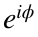. This leads to a new version of z, denoted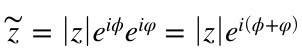.
Let's look at the mean real and imaginary components of the velocity before and after this rotation.
format short %ask Matlab to not print too many digits
mean(cv(:,4))
ans = 20.7858 - 11.8093i
mean(cv(:,4)*exp(-1i*phi))
ans = 23.9063 + 0.0000i
After the rotation, the mean of the cross-stream velocity is zero, as expected.
The combination exp(1i*phi)) occurs frequently. In jLab there is a shorthand for this, called rot.
mean(cv(:,4)*rot(-phi))
ans = 23.9063 + 0.0000i
It is useful to approach this rotation from another direction. As we recalled in class, the counterclockwise rotation matrix, which I typically denote by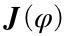, is given byTo rotate a vector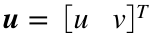, where T denotes the transpose, through the angle φ, we left-multiply u by.Since we want to multiply through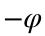, the rotation matrix is given by
J=[cos(phi) -sin(-phi); sin(-phi) cos(phi)]
J = 2×2
0.8695 -0.4940
0.4940 0.8695
where we can omit the minus sign in the arguments of the cosines, since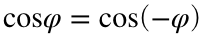. Then we carry out this matrix rotation at each time as follows:
cvr=zeros(size(cv(:,4)));
for i=1:length(cv)
uv=J*[real(cv(i,4)); imag(cv(i,4))];
cvr(i)=uv(1)+1i*uv(2);
end
mean(cvr)
ans = 23.9063 + 0.0000i
As you can see this does exactly the same thing as before. However, it's more cumbersome to work with the matrix multiplications. The convenience of rotations is one reason to prefer complex-valued notation for the velocity.

# Not the Mean Direction!

Note that the angle of the mean flow is not at all the same as the mean angle! If you think about it, it doesn't make sense to take the average of the instantaneous angle, as this is a 2πperiodic quantity. So if we use a term like ‘mean direction’, that is unclear.
What we want is the direction of the mean flow, not the mean of the direction. Here are some examples of why you don't want to take the mean of the direction.
ans = -29.0225
In this case, it is not too different from the direction of the mean flow.
However, if we rotate the velocity by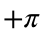/2, take the mean of the direction, and subtract π/2, we don't get the same answer!
ans = -30.0970
This is because the mean of the direction depends upon the choice of reference frame. This is a consequence of the fact that the angle is 2πperiodic.
The right way to find a ‘mean direction’ associated with a complex-valued number, or a vector, is to find the direction of the mean.

# Plotting the Rotated Velocity

In what follows we will work only with the velocities rotated by the angle, the direction of the mean flow at the deepest current meter as found above. The real and imaginary parts are now the downstream and cross-stream velocity components, respectively. To do this we redefine the velocity as
cv=m1244.cv*rot(-phi);
Next we will examine a time series plot of this rotated velocity.
clf,uvplot(yearfrac(num),vfilt(cv(:,4),24)),axis tight,
title(['Rotated Currents at ' int2str(depths(4)) ' m'])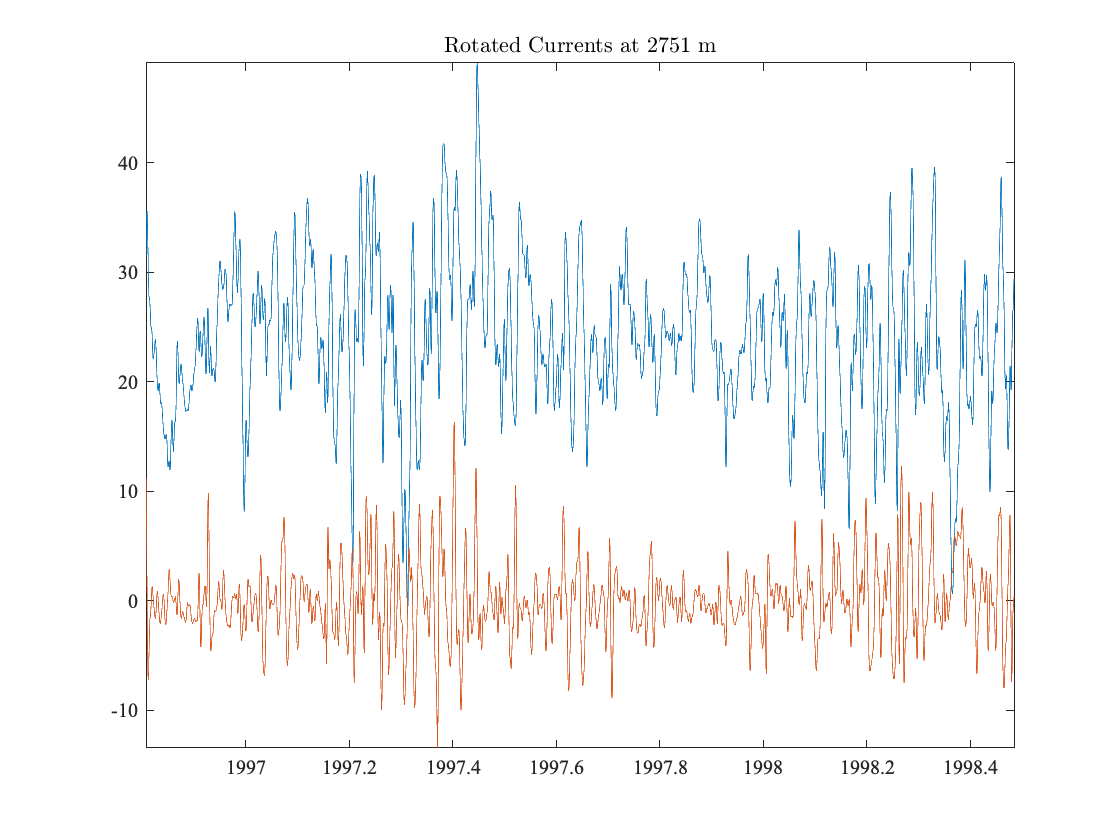The downstream and cross-stream components are distinctly different. The cross-stream flow oscillates about a mean of zero, which is by construction, and also presents higher-frequency variability than does the downstream flow. Or, perhaps more accuately, it appears that the cross-stream flow is lacking a low-frequency component that is present in the downstream flow. These different timescales between the two components is not what one would expect if the variability were entirely due to the advection of eddies past the mooring---the timescales in the that case would be the same. This will be examined in more detail later.

# Two-Dimensional Histograms

Next we will look at two-dimensional distributions of the velocity. Firstly, we plot the two-dimensional histogram at the deepest depth. Again, this is in the rotated frame such that the postive x-axis is in the direction of the time mean currents.
[mat,xmid,ymid]=...
twodhist(real(cv(:,4)),imag(cv(:,4)),[-50:50],[-50:50]);
clf,jpcolor(xmid,ymid,mat);
hold on,plot(mean(cv(:,4)),'wo','markerfacecolor','k')
colormap lansey, axis equal, axis(49*[-1 1 -1 1])
xtick([-40:10:40]),ytick([-40:10:40])
vlines(0,'w:'),hlines(0,'w:'),colorbar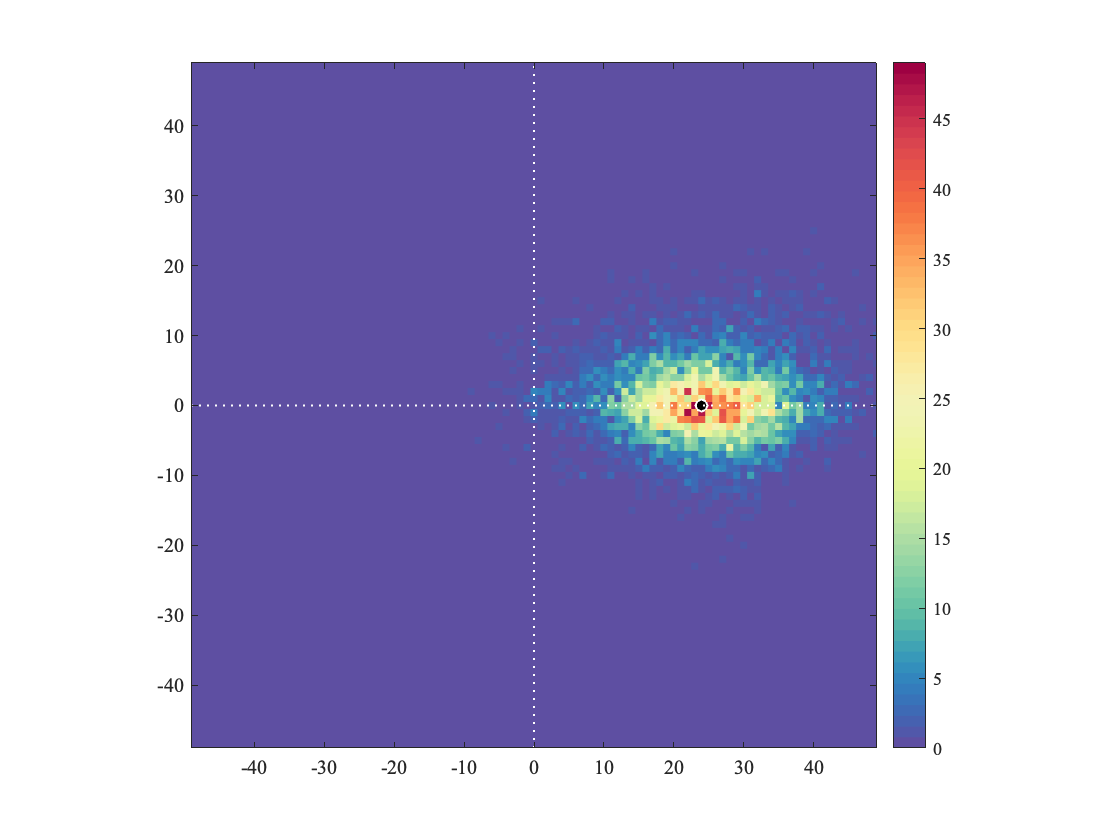The colorbar shows the number of observations within each bin. This plot shows that the histogram of the velocity has a roughly elliptical shape.
There are several things to note in the above code:
1. twodhist has been instructed to find the distribution of both uand v between -50 and 50 cm/s, with a bin size of one cm/s.
2. We are plotting the mean currents as a small circle. One trick I like, used here, is to set the edge and face color to be different, which helps with visibility.
3. lansey is my favorite colormap, included with jLab. It is more legible than jet and parula in my opinion. Type ‘lansey --f’ for a comparison.
4. xtick and ytick are self-explanatory jLab shorthands for setting the tick-mark locations.
5. hlines and vlines are simple jLab functions for plotting horizontal and vertical lines, respectively. Note that both of these functions support the linestyle syntax we discussed previously.
The very quick and dirty way to use twodhist is with three arguments. Here the third argument just tells the number of bins you want in the x- and y-directions, and the bins are chosen internally to be linearly spaced between maximum and minimum values.
[mat,xmid,ymid]=twodhist(real(cv(:,4)),imag(cv(:,4)),50);
clf,jpcolor(xmid,ymid,mat);
axis equal, axis(49*[-1 1 -1 1])
xtick([-40:10:40]),ytick([-40:10:40])
vlines(0,'w:'),hlines(0,'w:'),colorbar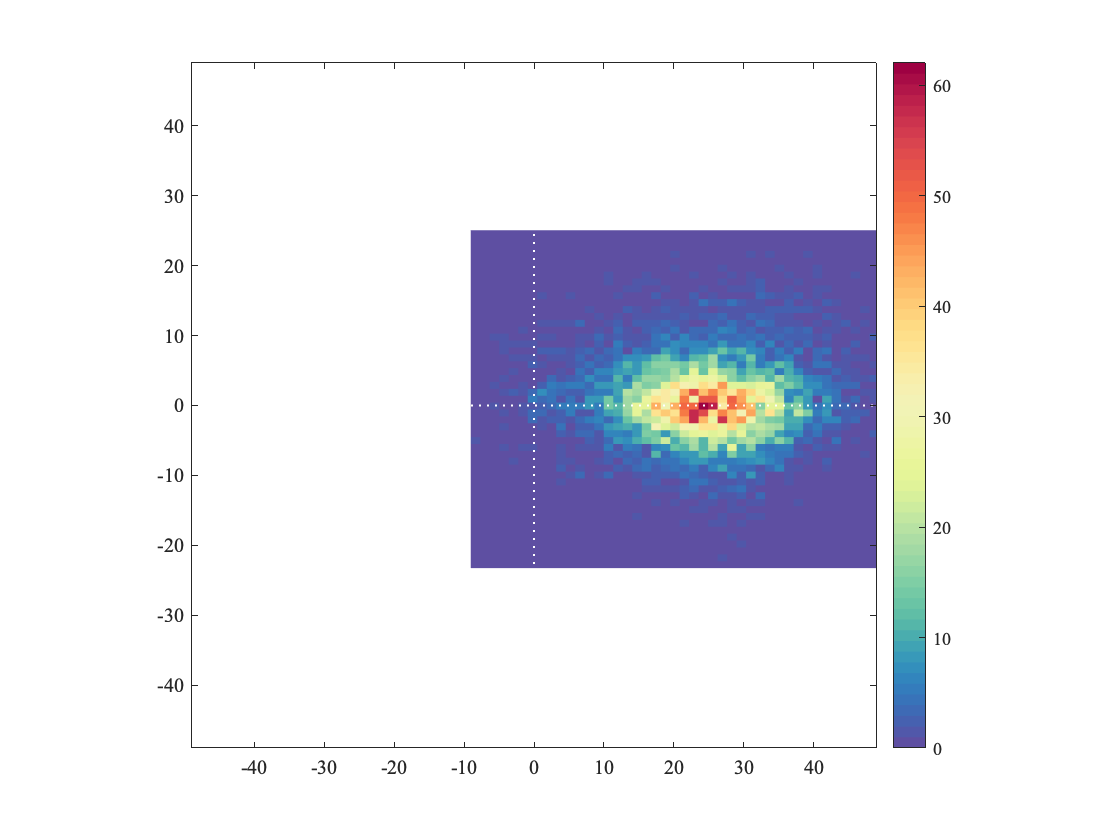Now we will compute a quantity called the variance ellipse. We will study this more in the lectures; here we will just get a feeling for how it works.
First we form the terms in the covariance matrix for the rotated velocities at the deepest depth. Then, we diagonalize the covariance matrix with a jLab routine called specdiag .
u=real(cv(:,4));u=u-mean(u);
v=imag(cv(:,4));v=v-mean(v);
cuu=mean(u.*u);
cvv=mean(v.*v);
cuv=mean(u.*v);
[a2,b2,theta]=specdiag(cuu,cvv,cuv);
Because we have both u and v, the second-order statistics of the velocity are not just the variances of u and v separately. One must also take into account their covariance.
The diagonalization of the covariance matrix expresses this same information in a different way. It will be shown in class that a2 and b2 are the squares of the semi-major and semi-minor axes of an ellipse, respectively, while theta is the orientation of the major axis of the ellipse with respect to the x-axis.
This ellipse is called the variance ellipse. Just as the variance is the fundamental second-order statistical quantity for a single or univariate ariance time series, such as u, the variance ellipse is the fundamental second-order statistical quantity for a pair of time series ().
[mat,xmid,ymid]=...
twodhist(real(cv(:,4)),imag(cv(:,4)),[-50:50],[-50:50]);
clf,jpcolor(xmid,ymid,mat),%caxis([1.75 2.2])
hold on,plot(mean(cv(:,4)),'wo','markerfacecolor','k')
colormap lansey, axis equal, axis(49*[-1 1 -1 1])
xtick([-40:10:40]),ytick([-40:10:40])
vlines(0,'w:'),hlines(0,'w:'),colorbar
[kappa,lambda]=ab2kl(sqrt(a2),sqrt(b2));
ellipseplot(kappa,lambda,theta,mean(cv(:,4)),'2G')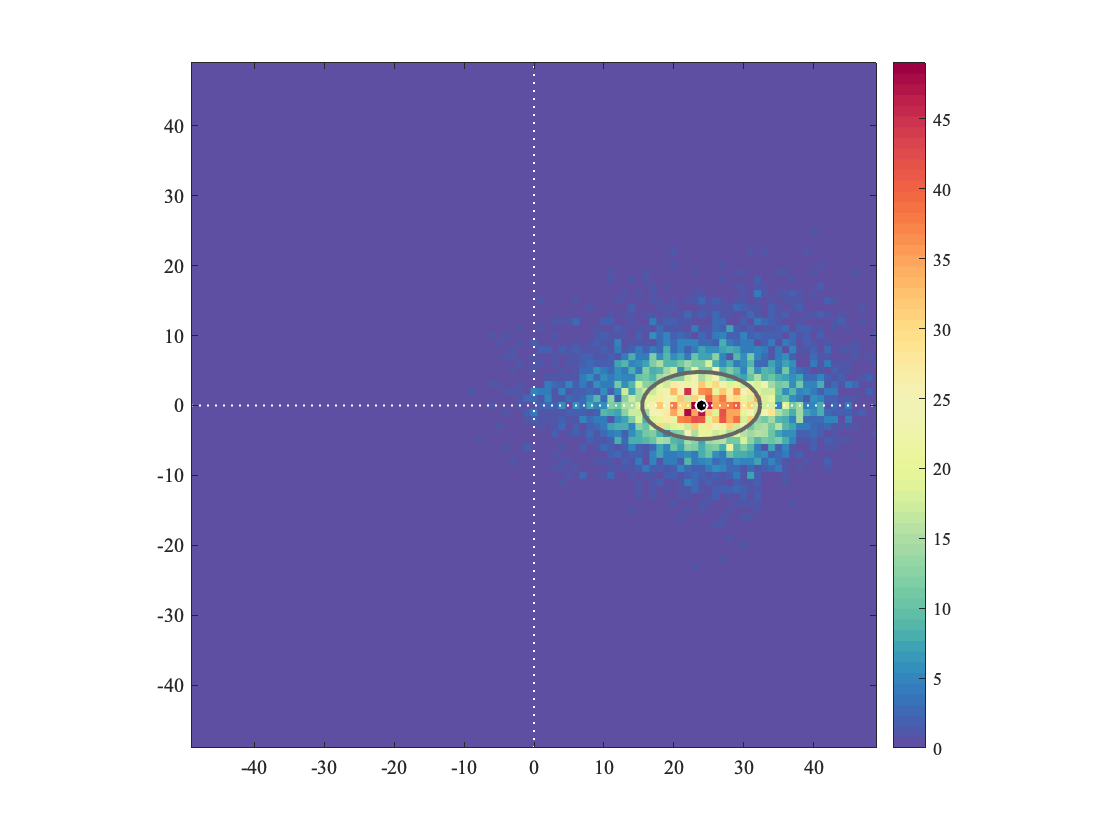You can see that the basic shape of the velocity distribution is indeed capture by the variance ellipse. Variance ellipses are a powerful tool for describing the basic features of velocity fluctuations, as we shall see.
In the above code, we have used jLab routine called ellipseplot to plot the ellipses. If you work with velocity data, please get to know this function, it will save you a lot of time.
Ellipseplot takes as its arguments not the ellipse semi-major and semi-minor axes a and b, but instead the related quantitieswhich describe the ellipse size and ellipse shape, respectively. κ is the ellipse root-mean-square axis length, while λ is a measure of the ellipse shape which, like the eccentricity, is equal to zero for a circle (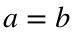) and one for a straight line (b=0). This conversion is carried out by the jLab routine ab2kl.
Next we will make the nicer version of the 2D histogram for all four depths, by just looping over the code blocks we have already used.
[kappa,lambda,theta]=vzeros(1,4);
for i=1:4
u=real(cv(:,i));u=u-mean(u);
v=imag(cv(:,i));v=v-mean(v);
[a2,b2,theta(i)]=specdiag(mean(u.*u),mean(v.*v),mean(u.*v));
[kappa(i),lambda(i)]=ab2kl(sqrt(a2),sqrt(b2));
end
clf
for i=1:4
subplot(2,2,i)
[mat,xmid,ymid]=...
twodhist(real(cv(:,i)),imag(cv(:,i)),[-50:50],[-50:50]);
jpcolor(xmid,ymid,mat);
hold on,plot(mean(cv(:,i)),'wo','markerfacecolor','k')
colormap lansey, axis equal, axis(49*[-1 1 -1 1])
xtick([-40:10:40]),ytick([-40:10:40])
vlines(0,'w:'),hlines(0,'w:'),
ellipseplot(kappa(i),lambda(i),theta(i),mean(cv(:,i)),'2w')
title(['Mooring 1244, ' num2str(depths(i)) ' m depth'])
end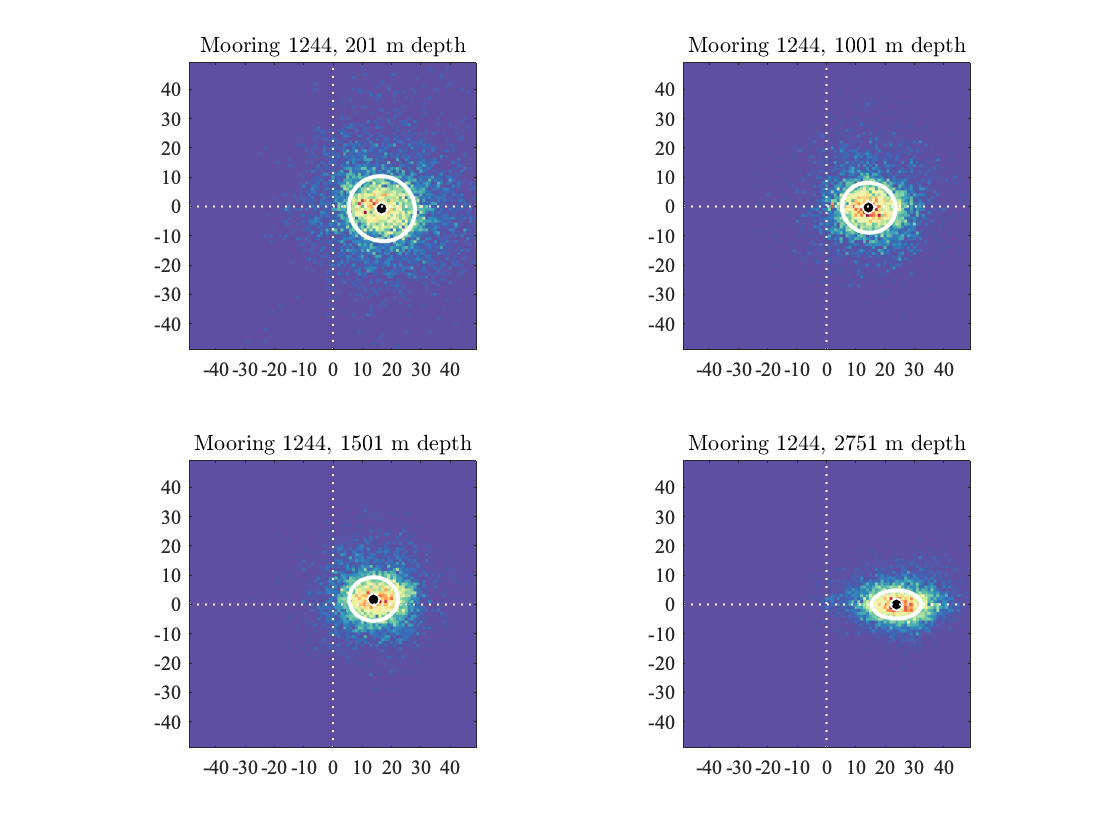This shows that as we proceed down in depth, the current variability becomes increasingly anisotropic, that is, lacking in isotropy. Another way to say this is that the velocity fluctuations become increasingly polarized with depth.
Note that the orientation of the variance ellipse at the deepest depth is virtually identical to the orientation of the mean flow. That is often but not always the case.

# Velocity vs. Temperature Fluctuations

Let's move on now to take a look at the co-variability of temperature and velocity. First we will make a simple time series plot. This is just the same rotated velocity signal we've been looking at, with temperature at the same depth plotted underneath it.
clf,subplot(2,1,1),uvplot(yearfrac(num),vfilt(cv(:,4),24)),axis tight,
title(['Rotated Currents and Temperature at ' int2str(depths(4)) ' m'])
subplot(2,1,2),plot(yearfrac(num),vfilt(t(:,4),24)),axis tight,
packfig(2,1,'rows')